Let P be a point distinct of the centroid G and not lying on a sideline of ABC. Let K1 = pK(G, P) be the isotomic pivotal cubic with pivot P and K2 = pK(P, G) the pivotal cubic with pivot G. Several already mentioned such cubics are given in the following tables together with some other remarkable ones.K1 P remarks / centers on the cubic K007 X69 Lucas cubic K008 X316 Droussent cubic, the only circular cubic K034 X75 Spieker perspector cubic K045 X264 Euler perspector cubic K092 X11057 the only equilateral cubic K133 X309 K141 X76 K146 X3 K154 X322 K170 X4 K200 X8 Soddy-Gergonne-Nagel cubic K235 X14615 Yiu cubic K240 X892 K242 X99 K254 X314 K264a X298 K264b X299 K279 X3260 K311 X320 Parry cubic K355 X511 K371 X11055 K377 X14558 isotomic McCay cubic K455 X319 K605 X304 K610 X286 K611 X340 K659 X6 K860 X30 K985 X1966 K998 X1965 K1000 X5207 K1002 X4645 K1004 X662 K1037 X18906 K1053a X621 K1053b X622 K1078 X7
 K2 P remarks / centers on the cubic K002 X6 Thomson cubic K043 X187 Droussent medial cubic, the only circular cubic K168 X3 K177 X32 K237 X1l5 K251 X238 K252 X1691 K253 X2092 K284 X574 K321 X5019 K341a X15 K341b X16 K345 X37 K357 X511 K363 X1 K453 X44 K472 X30 K485 X14537 the only equilateral cubic K489 X3003 K612 X216 K637 X1100 K663 X4 K671 X53 K836 X39 K856 X323 K857 X394 K879 X393 K924 X800 K965 X57 K1012 X3094 K1035 X18904 K1036 X18905 K1045 X3926 K1148 X519 K1149 X4908 K1162 X1184Remarks : • the isotomic transform of K2 is pK(tP, tP), see CL007. • the complement of K1(P) is K2(cP) and the anticomplement of K2(P) is K1(aP). • each cubic K1 corresponds to a central cubic pK(cP, atP) with center ctP (see Special Isocubics, § 3.1.3 and Central Cubics).Locus properties All these cubics are related to orthologic triangles. Indeed, • pK(G, P) is the locus of point M such that the cevian triangle of M is orthologic to the pedal triangle of gtP or to the antipedal triangle of tP, • pK(P, G) is the locus of point M such that the anticevian triangle of M is orthologic to the pedal triangle of gtaP or to the antipedal triangle of taP, where the prefixes g, t, a, c denote isogonal, isotomic, anticomplement, complement respectively. In all cases, the locus of one center of orthology is a central cubic circumscribing the triangle ABC for one center and the pedal/antipedal related triangle for the other center. Note that these cubics are pivotal cubics with respect to the corresponding triangle. The asymptotes of one cubic are the lines passing through the center of the cubic and the midpoints of the corresponding other triangle. Example 1 : the Lucas cubic K007 = pK(X2, X69) is the locus of point M such that the cevian triangle of M is orthologic to the medial and antimedial triangles. One of the centers of orthology lies on the Darboux cubic K004 in both cases, the other on the complement or the anticomplement of K004. Example 2 : the Thomson cubic K002 = pK(X6, X2) is the locus of point M such that the anticevian triangle of M is orthologic to the medial and antimedial triangles. One of the centers of orthology lies on the Darboux cubic K004 in both cases, the other on the complement or the anticomplement of K004. *** Other properties in the page K636.Pencils of cubics For a given point P, let us consider the pencil F of cubics generated by K1 and K2. Each cubic of F contains A, B, C, G (counted twice), P and three (not always real) points S1, S2, S3 on the Steiner ellipse. All these cubics (except K4, see below) are tangent at G to the line GP. F always contains several other remarkable cubics namely : – one decomposed cubic which is the union of the Steiner ellipse and the line GP. – a third pK which is K3 = pK(tcP, tcP), a member of the class CL007 . tcP is the isotomic conjugate of the complement of P. – a nodal cubic K4 with node G. The nodal tangents are parallel to the asymptotes of the circumconic which is the isotomic transform of the line GP. They are perpendicular when P lies on the line GK. – a cubic K5 with concurring asymptotes at X, the homothetic of P under h(G, 1/3). These asymptotes are parallel to the medians of ABC.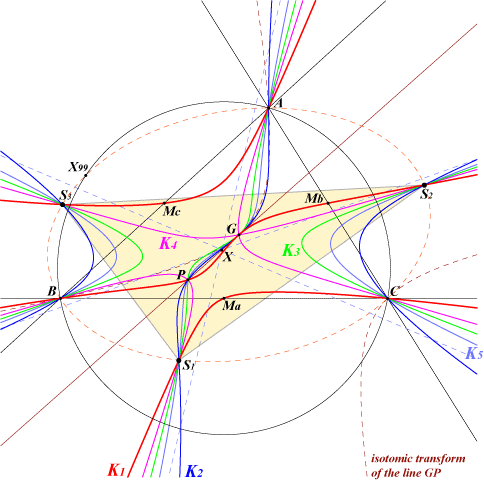The figure shows the case P = X(69) where K1 is the Lucas cubic. K2 is pK(X69, X2) passing through X(2), X(69), X(2996), K3 is K184 = pK(X76, X76), K4 has perpendicular nodal tangents parallel to the asymptotes of the Kiepert hyperbola, K5 has three real asymptotes concurring at X = X(21356).Moreover, the pencil F contains a circular cubic and an equilateral cubic if and only if P lies at infinity or on the line L passing through G and X(187), the inverse of X(6) in the circumcircle. F with P at infinity This first case is not very interesting since the circular cubic and the equilateral cubic decompose and coincide with K5. These are the union of the line at infinity and the circumconic which is the isotomic transform of the line GP. Nevertheless, we observe that K2 is now the complement of K1. K4 becomes an isotomic cK0 (see Special Isocubics, §8) with root R at infinity. R is the infinite point of the polar line of P in the Steiner ellipse. The pivotal conic is a parabola. F with P on L Let us now take P on the line L. This line contains X(i) for i = 2, 187, 316, 598, 625, 1153, 1383, 3849, 3972, 5215, 5475, 5569, 6031, 6032, 7603, 7737, 7761, 7771, 7804, 7831, 7853, 7898, 7934, 7937, 8176, 8182, 8785, 9829, 10150, 10162, 10163, 10173, 11057, 11614, 11655, 14537, 14712, 14762, 14907, 14976, 15810, 15820, 15822, 15880, 16275, 19569, 21843, 23297, 23334, 24638, 26079, 26145, 26276, 26613, 28440, 28727, 29543, 29804, 30021, 30862, 30932, 30999, 31132, 31173, 31275, 31415, 32827, 34205, 34245, 37809, 39602, 40344. P is defined by GP = k GX(187) (vectors) where k is a real number or infinity.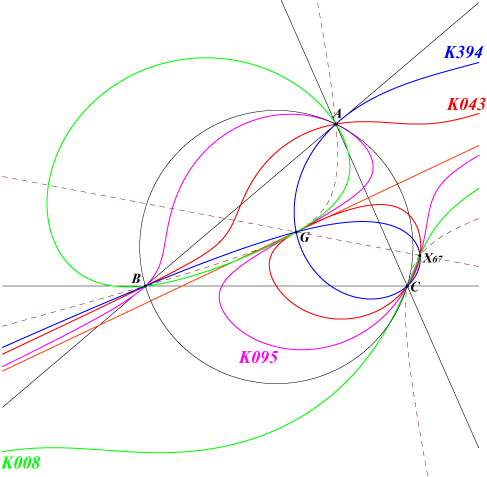Circular cubics F contains a circular cubic K6 which always passes through X(67) and X(524). These cubics form a pencil generated by the Droussent cubic K008 and the Droussent medial cubic K043 obtained with P = X(316) and P = X(187) respectively. The only nodal cubic of the pencil is K394 obtained when P is the reflection of G in X(187). When P = G (limit case), we obtain K095, a central focal orthopivotal cubic. When P is the infinite point of L, K6 decomposes into the line at infinity and the circumconic passing through X(2), X(67) and X(599). The third point on GX(67) is the point E1 such that GE1 = (k-2)/2 GX(67). The third point on GX(524) is the point E2 such that GE2 = (–1+2/k) GX(6).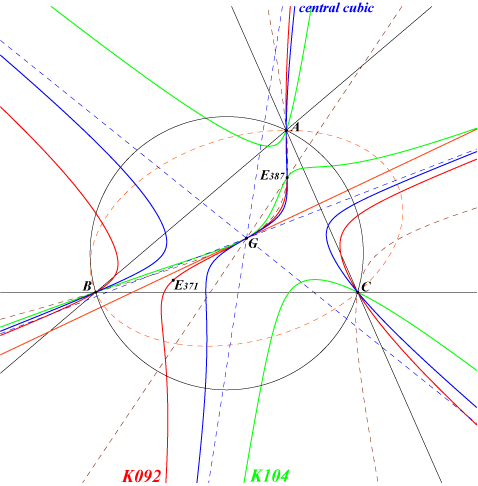Equilateral cubics Let X(11057) be the intersection of the lines GX(187) and X(30)X(76) and X(11058) its isotomic conjugate. F contains an equilateral cubic K7 which passes through X(11058) and three fixed points at infinity – those of K092 = pK(X2, X(11057)). The three asymptotes form an equilateral triangle whose centroid is a point of the Euler line. They concur if and only if k = 0 (limit case), in which case we obtain a central cubic with center G. These cubics K7 also form a pencil of cubics generated by K092 and the cubic decomposed into the line at infinity and the circumconic passing through X(2), X(67), X(599) and X(11058) (the same conic as above). The pencil also contains K104 and the central cubic with center G.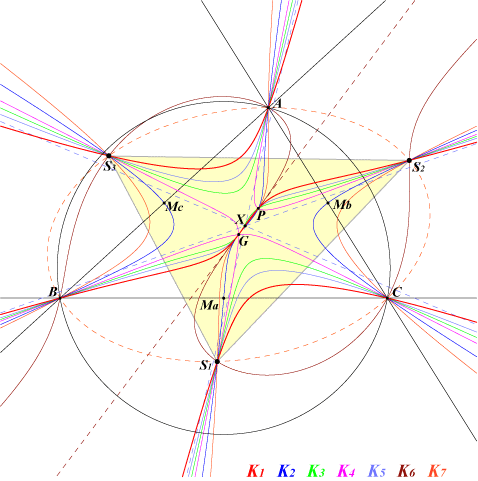The pencil F with P = X(598) X(598) is the second intersection of the line GX(187) with the Kiepert hyperbola. This gives seven distinct cubics passing through G and X(598). K1 = pK(X2, X598) contains X(599), X(1992). K2 = pK(X598, X2). K3 = pK(tcP, tcP) with tcP = 1/[(b^2+c^2+4a^2)(2b^2+2c^2-a^2)] : : . K4 nodal cubic K5 contains X(76). K6 contains X(67), X(524). K7 contains H.Equilateral triangle S1S2S3 There is a unique isotomic pK meeting the Steiner ellipse at the vertices of an equilateral triangle S1S2S3. This is K371 = pK(X2, X(11055)) where X(11055) is the homothetic of X(76) under h(G, –3). It follows that K371 = K1 and pK(X2, X(11055)) = K2 generate a pencil F of cubics which all meet the Steiner ellipse at A, B, C, S1, S2, S3. These points S1, S2, S3 lie on the circle with center E374 passing through X(671) which is its fourth intersection with the Steiner ellipse. X(671) is the antipode of the Steiner point X(99) on the Steiner ellipse and E374 is the reflection of X(76) in G. This circle is the reflection in G of the circle with center X(76) passing through X(99). See "A Morley Configuration" in the Downloads page.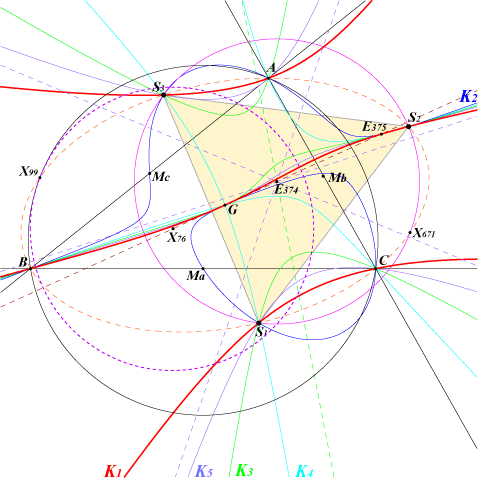Compare K371 and K089, an isotomic nK whose root is X(11055) which also meets the Steiner ellipse at the vertices of an equilateral triangle. Since X(11055) does not lie on the line GX(187), the pencil F does not contain a circular cubic nor an equilateral cubic. K3 is the cubic with pole and pivot tE385, the isotomic conjugate of E385 = anticomplement of X(11055). K4 is the nodal cubic. K5 has three real asymptotes parallel to the medians and concurring at E374, the centroid of S1S2S3. Remark : K3 is the locus of the poles Ω and also of the pivots P of all pK(Ω, P) passing through these points S1, S2, S3. In other words, for any point Ω (resp. P) on K3, there is a pK with pole Ω, pivot P, which contains these points.